# Supported Operations¶

This page lists and describes all supported image operations, and is mainly intended as a quick preview of the available functionality.

Category Available Operations
Mirroring FlipX FlipY
Rotating Rotate90 Rotate270 Rotate180 Rotate
Shearing ShearX ShearY
Distorting ElasticDistortion
Scaling and Resizing Scale Zoom Resize
Cropping Crop CropNative CropSize CropRatio RCropRatio
Conversion ConvertEltype
Information Layout SplitChannels CombineChannels PermuteDims Reshape
Utility Operations NoOp CacheImage Either

## Affine Transformations¶

A good portion of the provided operations fall under the category of affine transformations. As such, they can be described using what is known as an affine map, which are inherently compose-able if chained together. However, utilizing such a affine formulation requires (costly) interpolation, which may not always be needed to achieve the desired effect. For that reason do some of the operations below also provide a special purpose implementation to produce their specified result. Those are usually preferred over the affine formulation if sensible considering the complete pipeline.

### Mirroring¶

class FlipX

Reverses the x-order of each pixel row. Another way of describing it would be to mirror the image on the y-axis, or to mirror the image horizontally.

julia> FlipX()
Flip the X axis

julia> FlipX(0.3)
Augmentor.Either (1 out of 2 operation(s)):
- 30% chance to: Flip the X axis
- 70% chance to: No operation

Input Output for FlipX()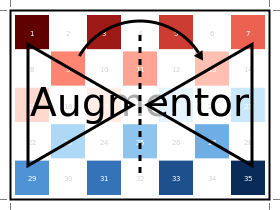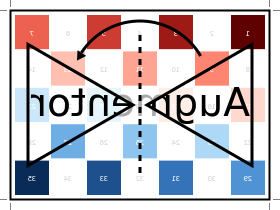class FlipY

Reverses the y-order of each pixel column. Another way of describing it would be to mirror the image on the x-axis, or to mirror the image vertically.

julia> FlipY()
Flip the Y axis

julia> FlipY(0.3)
Augmentor.Either (1 out of 2 operation(s)):
- 30% chance to: Flip the Y axis
- 70% chance to: No operation

Input Output for FlipY()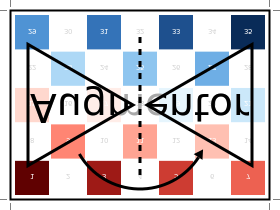### Rotating¶

class Rotate90

Rotates the image upwards 90 degrees. This is a special case rotation because it can be performed very efficiently by simply rearranging the existing pixels. However, it is generally not the case that the output image will have the same size as the input image, which is something to be aware of.

julia> Rotate90()
Rotate 90 degree

julia> Rotate90(0.3)
Augmentor.Either (1 out of 2 operation(s)):
- 30% chance to: Rotate 90 degree
- 70% chance to: No operation

Input Output for Rotate90()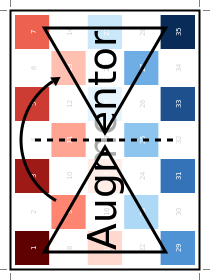class Rotate180

Rotates the image 180 degrees. This is a special case rotation because it can be performed very efficiently by simply rearranging the existing pixels. Furthermore, the output image will have the same dimensions as the input image.

julia> Rotate180()
Rotate 180 degree

julia> Rotate180(0.3)
Augmentor.Either (1 out of 2 operation(s)):
- 30% chance to: Rotate 180 degree
- 70% chance to: No operation

Input Output for Rotate180()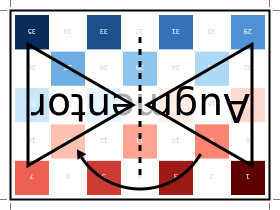class Rotate270

Rotates the image upwards 270 degrees, which can also be described as rotating the image downwards 90 degrees. This is a special case rotation, because it can be performed very efficiently by simply rearranging the existing pixels. However, it is generally not the case that the output image will have the same size as the input image, which is something to be aware of.

julia> Rotate270()
Rotate 270 degree

julia> Rotate270(0.3)
Augmentor.Either (1 out of 2 operation(s)):
- 30% chance to: Rotate 270 degree
- 70% chance to: No operation

Input Output for Rotate270()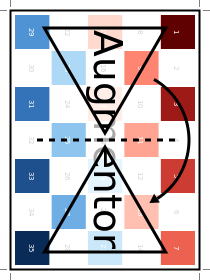class Rotate

Rotate the image upwards for the given degrees. This operation can only be described as an affine transformation and will in general cause other operations of the pipeline to use their affine formulation as well (if they have one).

In contrast to the special case rotations outlined above, the type Rotate can describe any arbitrary number of degrees. It will always perform the rotation around the center of the image. This can be particularly useful when combining the operation with CropNative.

julia> Rotate(15)
Rotate 15 degree

Input Output for Rotate(15)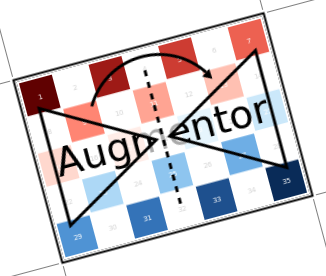It is also possible to pass some abstract vector to the constructor, in which case Augmentor will randomly sample one of its elements every time the operation is applied.

julia> Rotate(-10:10)
Rotate by θ ∈ -10:10 degree

julia> Rotate([-3,-1,0,1,3])
Rotate by θ ∈ [-3, -1, 0, 1, 3] degree

Input Sampled outputs for Rotate(-10:10)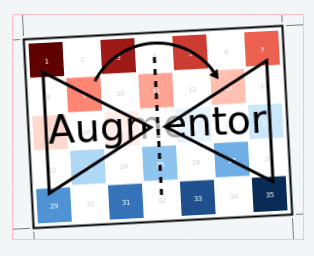### Shearing¶

class ShearX

Shear the image horizontally for the given degree. This operation can only be described as an affine transformation and will in general cause other operations of the pipeline to use their affine formulation as well (if they have one).

It will always perform the transformation around the center of the image. This can be particularly useful when combining the operation with CropNative.

julia> ShearX(10)
ShearX 10 degree

Input Output for ShearX(10)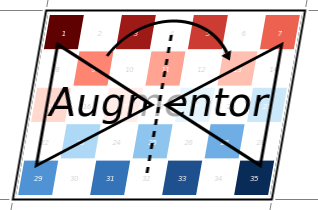It is also possible to pass some abstract vector to the constructor, in which case Augmentor will randomly sample one of its elements every time the operation is applied.

julia> ShearX(-10:10)
ShearX by ϕ ∈ -10:10 degree

julia> ShearX([-3,-1,0,1,3])
ShearX by ϕ ∈ [-3,-1,0,1,3] degree

Input Sampled outputs for ShearX(-10:10)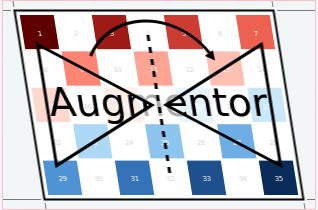class ShearY

Shear the image vertically for the given degree. This operation can only be described as an affine transformation and will in general cause other operations of the pipeline to use their affine formulation as well (if they have one).

It will always perform the transformation around the center of the image. This can be particularly useful when combining the operation with CropNative.

julia> ShearY(10)
ShearY 10 degree

Input Output for ShearY(10)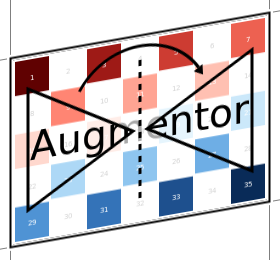It is also possible to pass some abstract vector to the constructor, in which case Augmentor will randomly sample one of its elements every time the operation is applied.

julia> ShearY(-10:10)
ShearY by ψ ∈ -10:10 degree

julia> ShearY([-3,-1,0,1,3])
ShearY by ψ ∈ [-3, -1, 0, 1, 3] degree

Input Sampled outputs for ShearY(-10:10)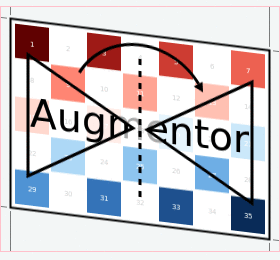### Scaling¶

class Scale

Multiplies the image height and image width by individually specified constant factors. This means that the size of the output image depends on the size of the input image.

julia> Scale(0.9,0.5)
Scale by 0.9×0.5

Input Output for Scale(0.9,0.5)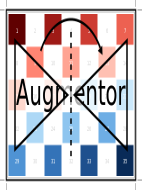In the case that only a single scale factor is specified, the operation will assume that the intention is to scale all dimensions uniformly by that factor.

julia> Scale(1.2)
Scale by 1.2×1.2

Input Output for Scale(1.2)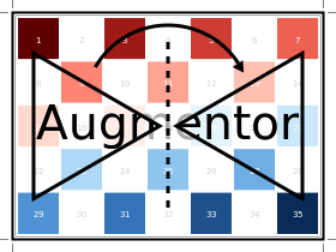It is also possible to pass some abstract vector(s) to the constructor, in which case Augmentor will randomly sample one of its elements every time the operation is applied.

julia> Scale([1.1, 1.2], [0.8, 0.9])
Scale by I ∈ {1.1×0.8, 1.2×0.9}

julia> Scale([1.1, 1.2])
Scale by I ∈ {1.1×1.1, 1.2×1.2}

julia> Scale(0.9:0.05:1.2)
Scale by I ∈ {0.9×0.9, 0.95×0.95, 1.0×1.0, 1.05×1.05, 1.1×1.1, 1.15×1.15, 1.2×1.2}

Input Sampled outputs for Scale(0.9:0.05:1.3)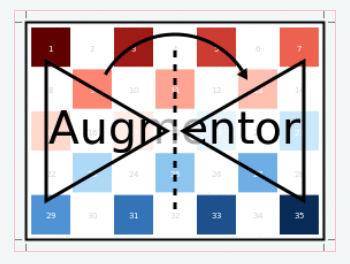class Zoom

Multiplies the image height and image width by individually specified constant factors. In contrast to Scale, the size of the input image will be preserved. This is useful to implement a strategy known as “scale jitter”.

julia> Zoom(1.2)
Zoom by 1.2×1.2

Input Output for Zoom(1.2)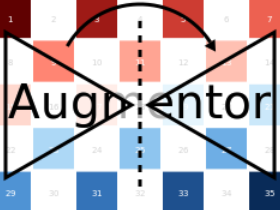It is also possible to pass some abstract vector to the constructor, in which case Augmentor will randomly sample one of its elements every time the operation is applied.

julia> Zoom([1.1, 1.2], [0.8, 0.9])
Zoom by I ∈ {1.1×0.8, 1.2×0.9}

julia> Zoom([1.1, 1.2])
Zoom by I ∈ {1.1×1.1, 1.2×1.2}

julia> Zoom(0.9:0.05:1.2)
Zoom by I ∈ {0.9×0.9, 0.95×0.95, 1.0×1.0, 1.05×1.05, 1.1×1.1, 1.15×1.15, 1.2×1.2}

Input Sampled outputs for Zoom(0.9:0.05:1.3)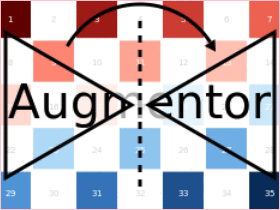## Distorting¶

class ElasticDistortion
Input Sampled outputs for ElasticDistortion(15,15,0.1)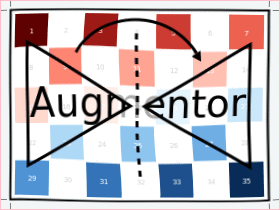Input Sampled outputs for ElasticDistortion(10,10,0.2,4,3,true)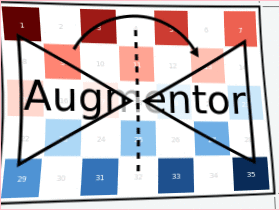## Resizing and Subsetting¶

The process of cropping is useful to discard parts of the input image. To provide this functionality lazily, applying a crop introduces a layer of representation called a “view” or SubArray. This is different yet compatible with how affine operations or other special purpose implementations work. This means that chaining a crop with some affine operation is perfectly fine if done sequentially. However, it is generally not advised to combine affine operations with crop operations within an Either block. Doing that would force the Either() to trigger the eager computation of its branches in order to preserve type-stability.

### Cropping¶

class Crop

Crops out the area of the specified pixel dimensions starting at a specified position, which in turn denotes the top-left corner of the crop. A position of x = 1, and y = 1 would mean that the crop is located in the top-left corner of the given image

julia> Crop(1:10, 5:20)
Crop region 1:10×5:20

julia> Crop(5, 1, 20, 10)
Crop region 1:10×5:24

Input Output for Crop(70:140,25:155)class CropNative

Crops out the area of the specified pixel dimensions starting at a specified position. In contrast to Crop, the the position (1,1) is not located at the top left of the current image, but instead depends on the previous transformations. This is useful for combining transformations such as Rotation or ShearX with a crop around the center area.

julia> CropNative(1:10, 5:20)
Crop native region 1:10×5:20

Output for (Rotate(45), Crop(1:210,1:280)) Output for (Rotate(45), CropNative(1:210,1:280))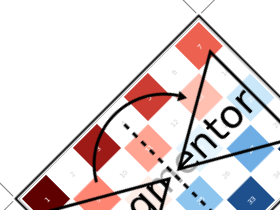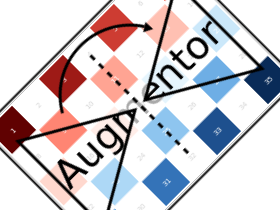class CropSize

Crops out the area of the specified pixel dimensions around the center of the given image.

julia> CropSize(45,250)
Crop a 45×250 window around the center

Input Output for CropSize(45,225)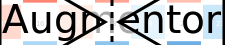class CropRatio

Crops out the biggest area around the center of the given image such that the output image satisfies the specified aspect ratio (i.e. width divided by height).

For example the operation CropRatio(1) would denote a crop for the biggest square around the center of the image, while CropRatio(16/9) would result in a rectangle with 16:9 aspect ratio.

julia> CropRatio(1)
Crop to 1:1 aspect ratio

julia> CropRatio(2.5)
Crop to 5:2 aspect ratio

Input Output for CropRatio(1)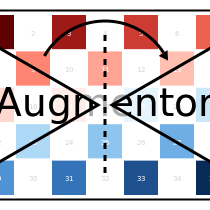class RCropRatio

Crops out the biggest possible area at some random position of the given image, such that the output image satisfies the specified aspect ratio (i.e. width divided by height).

For example the operation RCropRatio(1) would denote a crop for the biggest possible square. If there is more than one such square, then one will be selected at random.

julia> RCropRatio(1)
Crop random window with 1:1 aspect ratio

julia> CropRatio(2.5)
Crop random window with 5:2 aspect ratio

Input Sampled outputs for RCropRatio(1)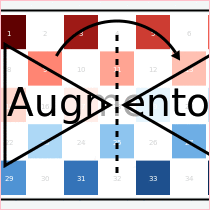### Resizing¶

class Resize

Transforms the image into a fixed specified pixel size. This operation does not take any measures to preserve aspect ratio of the source image. Instead, the original image will simply be resized to the given dimensions. This is useful when one needs a set of images to all be of the exact same size.

julia> Resize(30,40)
Resize to 30×40

Input Output for Resize(100,150)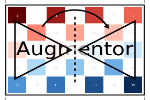## Conversion¶

class ConvertEltype

Convert the element type of the given array/image into the given eltype. This operation is especially useful for converting color images to grayscale (or the other way around). That said the operation is not specific to color types and can also be used for numeric arrays (e.g. with separated channels).

Note that this is an element-wise convert function. Thus it can not be used to combine or separate color channels. Use SplitChannels or CombineChannels for those purposes.

julia> op = ConvertEltype(Gray)
Convert eltype to Gray

julia> img = testpattern()
300×400 Array{RGBA{N0f8},2}:
[...]

julia> augment(img, op)
300×400 Array{Gray{N0f8},2}:
[...]

Input Output for ConvertEltype(GrayA)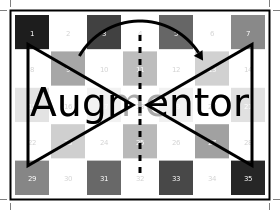## Information Layout¶

It is not uncommon that machine learning frameworks require the data in a specific form and layout. For example many deep learning frameworks expect the colorchannel of the images to be encoded in the third dimension of a 4-dimensional array.

Augmentor allows to convert from (and to) these different layouts using special operations that are mainly useful in the beginning or end of a augmentation pipeline.

### Color Channels¶

class SplitChannels

Separate the color channels of the given image into a dedicated array dimension. This will effectively create a new array dimension for the colors as the first dimension. In the case of greyscale images a singleton dimension will be created

This operation is mainly useful at the end of a pipeline in combination with PermuteDims in order to prepare the image for the training algorithm, which often requires the color channels to be separate.

julia> op = SplitChannels()
Split colorant into its color channels

julia> img = testpattern()
300×400 Array{RGBA{N0f8},2}:
[...]

julia> augment(img, op))
4×300×400 Array{N0f8,3}:
[...]

class CombineChannels

Combines the first dimension of a given array into a colorant of the specified type colortype. A separate color channel is also expected for Gray images.

The shape of the input image has to be appropriate for the given colortype, which also means that the separated color channel has to be the first dimension of the array. Use PermuteDims and/or Reshape if that is not the case.

This operation is mainly useful at the beginning of the pipline, if the colorchannels of the input images are separated.

julia> op = CombineChannels(RGB)
Combine color channels into colorant RGB{Any}

julia> A = rand(3, 10, 10) # random 10x10 RGB image
3×10×10 Array{Float64,3}:
[...]

julia> augment(A, op)
10×10 Array{RGB{Float64},2}:
[...]


### Array Shape¶

class PermuteDims

Permute the dimensions of the given array with the predefined permutation perm. This operation is particularly useful if the order of the dimensions needs to be different than the default “julian” layout.

More concretely, Augmentor expects the given images to be in vertical-major layout for which the colors are encoded in the element type itself. Many deep learning frameworks however require their input in a different order. For example it is not untypical that the color channels are expected to be encoded in the third dimension.

julia> op = PermuteDims(3,2,1)
Permute dimension order to (3,2,1)

julia> img = testpattern()
300×400 Array{RGBA{N0f8},2}:
[...]

julia> augment(img, PermuteDims(2,1))
400×300 Array{RGBA{N0f8},2}:
[...]

class Reshape

Reinterpret the shape of the given array of numbers or colorants. This is useful for example to create singleton dimensions that deep learning frameworks may need for colorless images, or for converting an image to a feature vector and vice versa.

Note that this operation has nothing to do with image resizing, but instead is strictly concerned with changing the shape of the array.

julia> Reshape(10,15)
Reshape array to 10×15

julia> op = Reshape(25)
Reshape array to 25-element vector

julia> A = rand(5,5)
5×5 Array{Float64,2}:
[...]

julia> augment(A, op)
25-element Array{Float64,1}:
[...]


## Utility Operations¶

Aside from “true” operations that specify some kind of transformation, there are also a couple of special utility operations used for functionality such as stochastic branching.

### Buffering¶

class CacheImage

Write the current state of the image into the working memory. Optionally a user has the option to specify a preallocated buffer to write the image into.

Even without a preallocated buffer it can be beneficial to cache the image in some situations. For example when chaining a number of affine transformations after an elastic distortion, because performing that lazily requires nested interpolation.

julia> CacheImage()
Cache into temporary buffer

julia> CacheImage(rand(5,5))
Cache into preallocated 5×5 Array{Float64,2}


### Identity Function¶

class NoOp

Pass the image along unchanged. Usually used in combination with Either to denote a “branch” that does not perform any computation.

julia> NoOp()
No operation


### Stochastic Branches¶

class Either

Allows for choosing between different operations at random when applied. This is particularly useful if one for example wants to first either rotate the image 90 degree clockwise or anticlockwise (but never both) and then apply some other operation(s) afterwards.

When compiling a pipeline, Either will analyze the provided operations in order to identify the most preferred way to apply the individual operation when sampled, that is supported by all given operations. This way the output of applying Either will be inferable and the whole pipeline will remain type-stable, even though randomness is involved.

By default each specified image operation has the same probability of occurrence. This default behaviour can be overwritten by specifying the chance manually.

julia> FlipX() * FlipY()
Augmentor.Either (1 out of 2 operation(s)):
- 50% chance to: Flip the X axis
- 50% chance to: Flip the Y axis

julia> Either(FlipX(), FlipY())
Augmentor.Either (1 out of 2 operation(s)):
- 50% chance to: Flip the X axis
- 50% chance to: Flip the Y axis

julia> Either((FlipX(), FlipY(), NoOp()), (1,1,2))
Augmentor.Either (1 out of 3 operation(s)):
- 25% chance to: Flip the X axis
- 25% chance to: Flip the Y axis
- 50% chance to: No operation

julia> Either(1=>FlipX(), 1=>FlipY(), 2=>NoOp())
Augmentor.Either (1 out of 3 operation(s)):
- 25% chance to: Flip the X axis
- 25% chance to: Flip the Y axis
- 50% chance to: No operation

julia> (1=>FlipX()) * (1=>FlipY()) * (2=>NoOp())
Augmentor.Either (1 out of 3 operation(s)):
- 25% chance to: Flip the X axis
- 25% chance to: Flip the Y axis
- 50% chance to: No operation